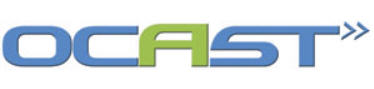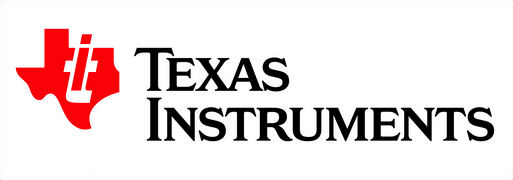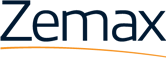## slider-spectroscopy2

Signal-to-Noise Ratio (SNR)

Ultimately, the ability of the spectrometer to make accurate measurements depends on the quality of the signal obtained from the detector and the subsequent electrical circuits. The signal-to-noise ratio (SNR) provides a measure of the signal quality. The SNR compares the average power available in the signal to the average power contained in the noise, which includes any signal from sources other than the target signal source. In a spectrometer, the desired signal consists of the optical power at a given wavelength directed by the diffraction grating (and by the DMD, in a DLP-based system) to the detector. The noise signal arises from a number of sources, both electrical and optical. The SNR is calculated according to:

SNR = Ī2sig / σ2n = (Ropt)2 / σ2 n1 + σ2 n2 + σ2 n3 +…… +σ2 nN                            (1)

where is the average optical power in the desired signal, and refers to the variance of the ith source of noise current, in A2, which describes the average power in a random signal based on Fourier Transform theory. To better understand the SNR equation, consider the two conditions in Figure SNR.1 below. In Figure SNR.1 (a), the signal current (numerator of SNR) and the noise current (denominator of SNR) are about equal – i.e., the SNR ≈ 1. One can see that in this condition, the spectrometer cannot separate the signal containing information about the sample from the noise signal, and thus the measurement provides no useful data. In Figure SNR.1 (b), the signal current greatly exceeds the noise current in magnitude, making the signal current easier to distinguish and separate from the noise. The measurement can now provide useful and reliable information about the sample.

(Figure SNR.1)

In order to improve the SNR in a spectrometer, the design choices must increase the power in the measurement signal while at the same time minimize the noise sources as much as possible.

Sources of Noise

Dark noise, also commonly referred to as thermal noise () arises from statistical changes in the number of electrons available to conduct current, even without light incident on the detector. Thermal energy provided by ambient heating generates the additional carriers that contribute to the noise current. For a detector connected to a simple resistor, the dark noise is given by

σ2th = 4kBT Δf / R

where kB is Boltzmann’s constant, T is the temperature in Kelvin, and Δf is the bandwidth of the electrical systems attached to the detector.

Shot noise () arises from the statistical variation in the number of photons incident on the detector, assuming all photons are converted to electrons that contribute to current. Thus, the magnitude of the shot noise depends on the optical power incident on the detector, according to

σ2s = 2qRoptΔf

where q is the charge on an electron, given by 1.602·10-19. The relative magnitude of the shot noise and thermal noise strongly depends on the ambient temperature, the value of at the detector, and the resistor used to convert the electrical current into a voltage for processing.

Additional electronic noise, sometimes referred to as the readout noise, arises from the circuitry directly behind the detector that provides the initial filtering and scaling of the signal. This noise can be modeled as an additional noise current () or by the use of a measurement called the Noise Figure (FN), defined as

FN = SNRin / SNR out

For linear electronic systems, FN is typically greater than 2, resulting in at least a 2x reduction in the SNR described by equation (1) at the detector.

Fixed pattern noise () arises from the variation in the response to incident light of the detectors in a detector array. The variation originates primarily from differences in quantum efficiency caused by differences in the aperture area and the thickness of the detectors that occurred during fabrication. Only spectrometers employing a linear detector array for discriminating between wavelengths suffers from this source of noise.

A source of optical noise arises from the generation of stray light within the optical system of the spectrometer. Imperfections in the diffraction grating’s structure and accidental routing of light from “off” mirrors on the DMD can result in light entering the detector through paths that contains no useful information of any kind. The stray light thus acts as a background illumination that must be exceeded before the output of a detector can be confidently identified as an information-carrying signal.

Improving SNR

Several methods of spectrometer design and measurement, based on the nature of the noise sources, can improve the SNR of the spectrometer and lead to higher quality measurements.

Increasing the throughput of the spectrometer’s optical system, an issue addressed in several of the preceding sections, increases the signal power available at the detector. Although also increases as the optical power increases, the signal power increases as and the noise increase only as , and thus the SNR increases overall. Higher also reduces the time over which optical power is collected, called the integration time, and thus reduces the contribution of to the total noise power, which also increases the SNR.

The use of holographic gratings, which have much fewer imperfections than ruled gratings, can reduce the stray light generated by the optical system. For this reason, holographic gratings are commonly used in UV spectrometers that suffer signal losses in the optical system due to absorption and are thus more susceptible to noise from stray light.

A thermo-electric cooler (TEC) attached to any detector reduces the effective temperature of the detector and thus can reduce the impact of on the SNR. Using a TEC proves especially effective in the case of low optical signal power incident on the detector, as the detector signal is integrated over a long period of time in order to increase the total optical signal power that is collected. Since this process integrates the noise currents as well, the integrated noise power can overwhelm the improved signal power. TEC systems reduce the noise to a sufficiently low level that longer integration times prove effective in increasing the SNR.

## clients logos table

 Optecks’ Research funded in part by:Optecks is an Authorized Design House at:Find us on zemax.com